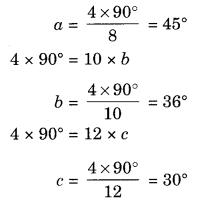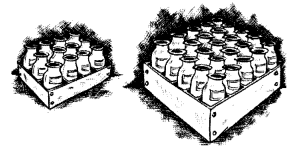# NCERT Solutions for Class 8 Maths Chapter 13 Direct and Inverse Proportions Ex 13.2

NCERT Solutions for Class 8 Maths Chapter 13 Direct and Inverse Proportions Ex 13.2

### NCERT Solutions for Class 8 Maths Direct and Inverse Proportions Exercise 13.2

Ex 13.2 Class 8 Maths Question 1.
Which of the following are in inverse proportion?
(i) The number of workers on a job and the time to complete the job.
(ii) The time taken for a journey and the distance travelled in a uniform speed.
(iii) Area of cultivated land and the crop harvested.

(iv) The time taken for a fixed journey and the speed of the vehicle.
(v) The population of a country and the area of land per person.
Solution:
(i) As the number of workers increase, the job will take less time to complete. Hence, they are inversely proportional.
(ii) For more time, more distance to travel. Hence, they are not inversely proportional.

(iii) More area of land cultivated, more crop to harvest. Hence, they are not inversely proportional.
(iv) If speed is increased, it will take less time to complete the fixed journey. Hence, they are inversely proportional.
(v) If the population of a country increases, then the area of land per person will be decreased. Hence, they are inversely proportional.

Ex 13.2 Class 8 Maths Question 2.
In a Television game show, the prize money of ₹ 1,00,000 is to be divided equally amongst the winners. Complete the following table and find whether the prize money given to an individual winner is directly or inversely proportional to the number of winners?

 Number of winners 1 2 4 5 8 10 20 The prize for each winner (in ₹) 1,00,000 50,000 – – – – –

Solution:
Let, the blank spaces be denoted by a, b, c, d and e.
So, we observe that 1 × 100,000 = 2 × 50,000
⇒ 1,00,000 = 1,00,000
Hence they are inversely proportional.
2 × 50,000 = 4 × aNumber of winners 1 2 4 5 8 10 20 The prize for each winner (in ₹) 1,00,000 50,000 25,000 20,000 12,500 10,000 5,000

Ex 13.2 Class 8 Maths Question 3.
Rehman is making a wheel using spokes. He wants to fix equal spokes in such a way that the angles between any pair of consecutive spokes are equal. Help him by completing the following table.Number of spokes 4 6 8 10 12 The angle between a pair of consecutive spokes 90° 60° – – –

(i) Are the number of spokes and the angle formed between the pairs of consecutive spokes in inverse proportion.
(ii) Calculate the angle between a pair of consecutive spokes on a wheel with 15 spokes.

(iii) How many spokes would be needed, if the angle between a pair of consecutive spokes is 40°?
Solution:
From the above table, we observe that
4 × 90° = 6 × 60°
360° = 360°
Thus the two quantities are inversely proportional.
Let the blank spaces be denoted by a, b, and c.
4 × 90° = 8 × aHence, the required table is

 Number of spokes 4 6 8 10 12 The angle between a pair of consecutive spokes 90° 60° 45° 36° 30°Ex 13.2 Class 8 Maths Question 4.
If a box of sweets is divided among 24 children, they will get 5 sweets each. How many would each get, if the number of the children is decreased by 4?
Solution:

 Number of children Number of Sweets 24 5 (24 – 4) or 20 a

We observe that on increasing the number of children, number of sweets got by each will be less. So, they are in inverse proportion.
x1y1 = x2y2
where x1 = 24, y1 = 5, x2 = 20
and y2 = a(let)
24 × 5 = 20 × a
a = 6
Hence, the required number of sweets = 6.

Ex 13.2 Class 8 Maths Question 5.
A farmer has enough food to feed 20 animals in his cattle for 6 days. How long would the food last if there were 10 more animals in his cattle?
Solution:
If the number of animals increases, then it will take fewer days to last.
Then the two quantities are in inverse proportions.

 Number of animals Number of days 20 6 (20 + 10) or 30 P

Let the required number of days be p.
x1y1 = x2y2
where x1 = 20, y1 = 6, x2 = 3
and y2 = p (let)
20 × 6 = 30 × p
p = 4
Hence the required number of days = 4.

Ex 13.2 Class 8 Maths Question 6.
A contractor estimates that 3 persons could rewire Jasminder’s house in 4 days. If, he uses 4 persons instead of three, how long should they take to complete the job?
Solution:
If the number of persons is increased, it will take less number of days to complete the job.
Thus, the two quantities are in inverse proportion.

 Number of persons Number of days 3 4 4 k

Let the required number of days be k.
x1y1 = x2y2
3 × 4 = 4 × k
k = 3 days.
Hence, the required number of days = 3.

Ex 13.2 Class 8 Maths Question 7.
A batch of bottles was packed in 25 boxes with 12 bottles in each box. If the same batch is packed using 20 bottles in each box, how many boxes would be filled?Solution:
If the number of bottles is increased then the required number of boxes will be decreased. Thus the two quantities are in inverse proportion.

 Number of boxes Number of bottles per box 25 12 x 20

Let the required number of boxes be x.
x1y1 = x2y2
25 × 12 = x × 20
x = 15
Hence, the required number of boxes = 15.

Ex 13.2 Class 8 Maths Question 8.
A factory requires 42 machines to produce a given number of articles in 63 days. How many machines would be required to produce the same number of articles in 54 days?
Solution:
If the number of machines is increased then less number of days would be required to produced the same number of articles.
Thus, the two quantities are in inverse proportion.

 Number of machines Number of days 42 63 x 54

Let the required number of machines be x.
x1y1 = x2y2
42 × 63 = x × 54
x = 49
Hence, the required number of machines is 49.

Ex 13.2 Class 8 Maths Question 9.
A car takes 2 hours to reach a destination by traveling at a speed of 60 km/h. How long will it take when the car travels at the speed of 80 km/h?
Solution:
On increasing the speed, it will take less time to travel a distance.
Thus the two quantities are in inverse proportions.

 Speed in km/h Time in hour 60 2 80 x

Let the required times be x hours.Ex 13.2 Class 8 Maths Question 10.
Two persons could fit new windows in a house in 3 days.
(i) One of the people fell ill before the work started. How long would the job take now?
(ii) How many persons would be needed to fit the windows in one day?
Solution:
On increasing the number of persons, less time will be required to complete a job.
Thus, the quantities are in inverse proportion.

 Number of persons Number of days 2 3 (i) 1(2 – 1) x (ii) y 1

(i) Let the required number of days be x.
x1y1 = x2y2
2 × 3 = 1 × x
x = 6
Hence, the required number of days = 6
(ii) Let the required number of persons be y.
x1y1 = x2y2
2 × 3 = y × 1
y = 6
Hence, the required number of persons = 6.

Ex 13.2 Class 8 Maths Question 11.
A school has 8 periods a day each of 45 minutes duration. How long would each period be, if the school has 9 periods a day, assuming the number of school hours to be the same?
Solution:
On increasing the duration of periods, the number of periods will be reduced.
Thus, the two quantities are in inverse proportion.

 Number of periods Duration of periods in minutes 8 45 9 x

Let the required duration of each period be x.
x1y1 = x2y2
8 × 45 = 9 × x
x = 40 minutes
Hence, the required duration of period = 40 minutes.## SabDekho

The Complete Educational Website